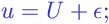# Glossary

#### Statistical Glossary

Additive error is the error that is added to the true value and does not depend on the true value itself. In other words, the result of the measurement is considered as a sum of the true value and the additive error:where

•is the result of a measurement of some quantity, say, weight;
•is the true value of the quantity;
•is the additive error, which is of the same physical dimension as the quantitybeing measured;

The model of additive errors is the most popular error model in statistics. In this model the additive error is assumed to be independent of the true value.

A related concept is multiplicative error .

Browse Other Glossary Entries

## Test Yourself

Planning on taking an introductory statistics course, but not sure if you need to start at the beginning? Review the course description for each of our introductory statistics courses and estimate which best matches your level, then take the self test for that course. If you get all or almost all the questions correct, move on and take the next test.

### Data Analytics

Considering becoming adata scientist, customer analyst or our data science certificate program?

Analytics Quiz

Statistics Quiz

### Statistics

Looking at statistics for graduate programs or to enhance your foundational knowledge?

Statistics 1 Quiz

Regression Quiz

Regression Quiz

### Biostatistics

Entering the biostatistics field? Test your skill here.

Biostatistics Quiz

Statistics 2 Quiz

### Stay Informed

Our Blog

Read up on our latest blogs

Certificates

Courses

Find the right course for you